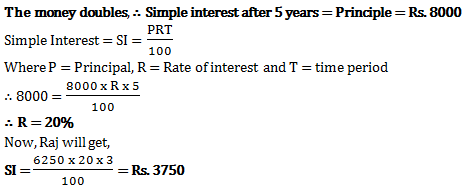# Simple Interest - Quantitative Aptitude (MCQ) questions for Q. 29349

Q.  Aman invests Rs. 8000 at some rate of interest. Being simple interest the money doubles in 5 years. Raj sees this and invests Rs. 6250 for 3 years at same rate of interest. How much interest does Raj get?
- Published on 11 Apr 17

a. Rs. 3750
b. Rs. 6250
c. Rs. 3125
d. Rs. 4250